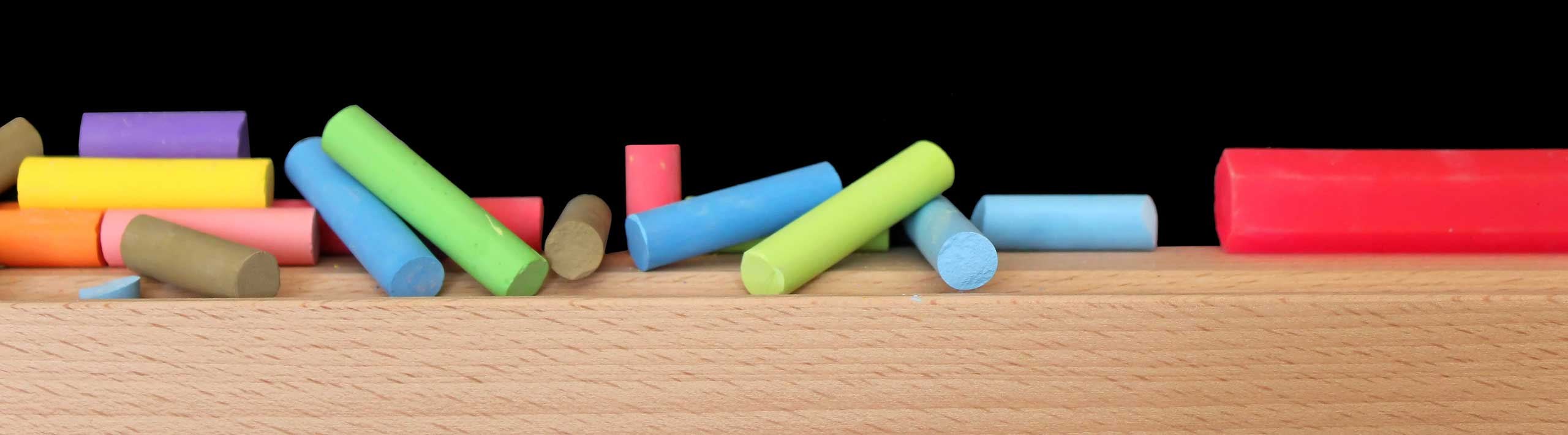# Introduction to Probability

## Probability of an Event

Probabilities are associated with experiments where the outcome is not known in advance or cannot be predicted. For example, if you toss a coin, will you obtain a head or tail? If you roll a die will obtain 1, 2, 3, 4, 5 or 6?
Probability measures and quantifies "how likely" an event, related to these types of experiment, will happen. The value of a probability is a number between 0 and 1 inclusive. An event that cannot occur has a probability (of happening) equal to 0 and the probability of an event that is certain to occur has a probability equal to 1.(see probability scale below).In order to quantify probabilities, we need to define the sample space of an experiment and the events that may be associated with that experiment.

## Sample Space and Events

The sample space is the set of all possible outcomes in an experiment.
Example 1: If a die is rolled, the sample space S is given by
S = {1,2,3,4,5,6}
Example 2: If two coins are tossed, the sample space S is given by
S = {HH,HT,TH,TT} , where H = head and T = tail.
Example 3: If two dice are rolled, the sample space S is given by
S = { (1,1),(1,2),(1,3),(1,4),(1,5),(1,6)
(2,1),(2,2),(2,3),(2,4),(2,5),(2,6)
(3,1),(3,2),(3,3),(3,4),(3,5),(3,6)
(4,1),(4,2),(4,3),(4,4),(4,5),(4,6)
(5,1),(5,2),(5,3),(5,4),(5,5),(5,6)
(6,1),(6,2),(6,3),(6,4),(6,5),(6,6) }

We define an event as some specific outcome of an experiment. An event is a subset of the sample space.
Example 4: A die is rolled (see example 1 above for the sample space). Let us define event E as the set of possible outcomes where the number on the face of the die is even. Event E is given by
E = {2,4,6}
Example 5: Two coins are tossed (see example 2 above for the sample space). Let us define event E as the set of possible outcomes where the number of head obtained is equal to two. Event E is given by
E = {(HT),(TH)}
Example 6: Two dice are rolled (see example 3 above for the sample space). Let us define event E as the set of possible outcomes where the sum of the numbers on the faces of the two dice is equal to four. Event E is given by
E = {(1,3),(2,2),(3,1)}

## How to Calculate Probabilities?

### 1 - Classical Probability Formula

It is based on the fact that all outcomes are equally likely.
 Total number of outcomes in E P(E)= ________________________________________________ Total number of outcomes in the sample space

Example 7: A die is rolled, find the probability of getting a 3.
The event of interest is "getting a 3". so E = {3}.
The sample space S is given by S = {1,2,3,4,5,6}.
The number of possible outcomes in E is 1 and the number of possible outcomes in S is 6. Hence the probability of getting a 3 is P(E) = 1 / 6.
Example 8: A die is rolled, find the probability of getting an even number.
The event of interest is "getting an even number". so E = {2,4,6}, the even numbers on a die.
The sample space S is given by S = {1,2,3,4,5,6}.
The number of possible outcomes in E is 3 and the number of possible outcomes in S is 6. Hence the probability of getting an even number is P(E) = 3 / 6 = 1 / 2.

### 2 - Empirical Probability Formula

It uses real data on present situations to determine how likely outcomes will occur in the future. Let us clarify this using an example
30 people were asked about the colors they like and here are the results:
 Color frequency red 10 blue 15 green 5

If a person is selected at random from the above group of 30, what is the probability that this person likes the red color?
Let event E be "likes the red color". Hence
 Frequency for red color P(E)= ________________________________________________ Total frequencies in the above table

= 10 / 30 = 1 / 3

Example 8: The table below shows students distribution per grade in a school.
 Grade frequency 1 50 2 30 3 40 4 42 5 38 6 50

If a student is selected at random from this school, what is the probability that this student is in grade 3?
Let event E be "student from grade 3". Hence
 Frequency for grade 3 P(E)= _______________________________________ Total frequencies

= 40 / 250 = 0.16